Dr. J's Maths.com
Where the techniques of Maths
are explained in simple terms.

Trigonometry - Measuring angles - calculating the three basic ratios.
Test Yourself 1.

HINTS: Always start by linking the angle to the side opposite with an arrow.

Use SOH CAH TOA but say it slowly and emphasise the sounds.

 Labelling sides. For each of the following 10 diagrams, copy the triangle and mark the hypotenuse, the side opposite the angle and the side adjacent to the angle. 1.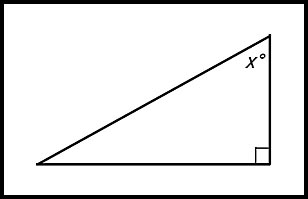2.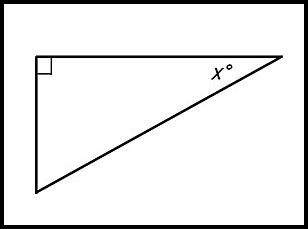3.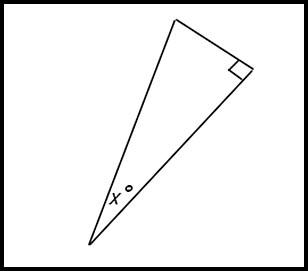4.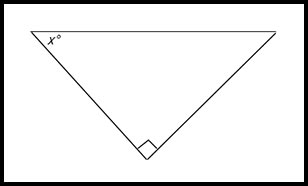5.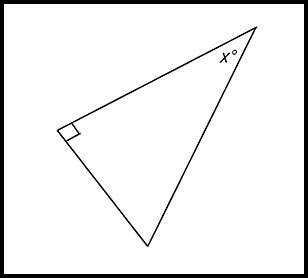6.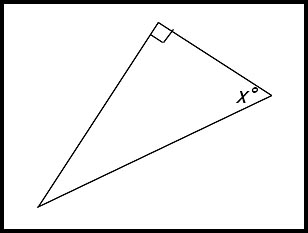7.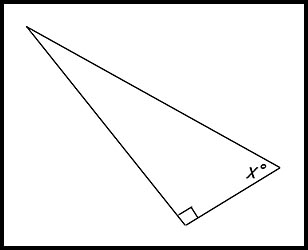8.9.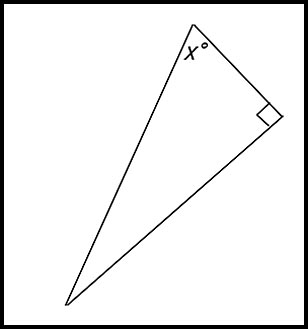10.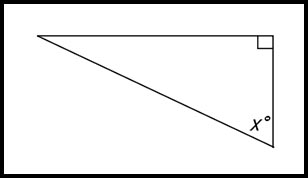Defining ratios. 11. What sides are required when calculating the cos of an angle? Answer.Need adjacent side and hypotenuse. 12. What sides are required when calculating the sin of an angle? Answer.Need opposite side and hypotenuse. 13. What is the difference between the ratio for cos and the ratio for sin? Answer.both need the hypotenuse but cos needs the adjacent side while sin needs the opposite side. 14. What is the difference between the ratio for tan and the ratio for cos? Answer.Tan needs the opposite side and cos needs the hypotenuse. Both ratios need the adjacent side. The ratios are in a different order - tan has opposite ÷ adjacent while cos has adjacent ÷ hypotenuse. Finding ratios. (use the diagram to write down the ratio indicated). 15. Write down the ratio of tan 39°.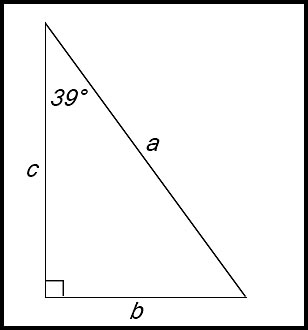Answer.b/c. 16. Write down the ratio of cos x°.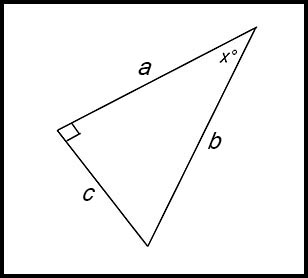Answer.a/b. 17. Write down the ratio of sin x°.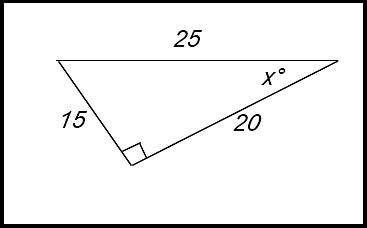Answer.3/5 or 0.6. 18. Write down the ratio of tan x°.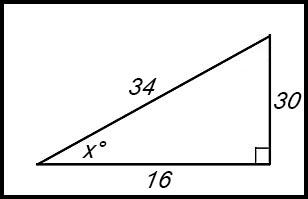Answer.5/4 or 1.25. 19. Write down the ratio of cos x°.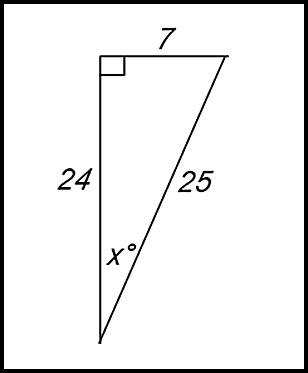Answer.24/25. 20. Write down the ratio of sin 58°.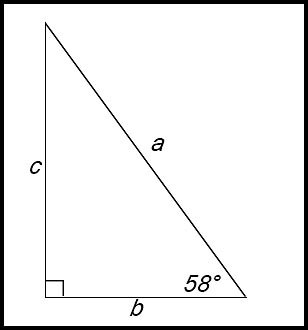Answer.c/a. 21.Write down the value of 12 tan x°.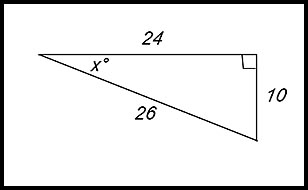Answer.5. 22. Write down the ratio of 4 sin x°.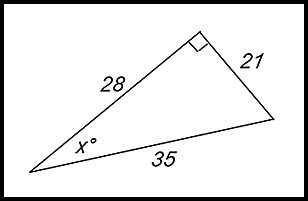Answer.12/5. Using a calculator to determine ratios. (answer to 4 decimal places). 23. Find the value of cos 63°. Answer.0.4540. 24. Find the value of tan 45°. Answer.1.0000. 25. Find the value of sin 89°. Answer.0.9998. 26. Find the value of cos 1°. Answer.0.9998. 27. Find the value of tan 80°. Answer.5.6713. 28. Find the value of sin 30°. Answer.0.5000. Miscellaneous. 29.If sin x has the its ratio value of, mark the angle x° and the right angle on the triangle. 30.If cos x has the its ratio value of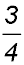, mark the angle x° and the right angle on the triangle.